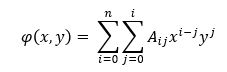# Grating with tilted phase front

This example file demonstrates the configuration of a grating surface using the XY Polynomial phase departure option to give an outgoing phase front with a 10 degree tilt applied along the y-axis.

Given the XY polynomial phase (OPD) departure of the form below, the A_11 coefficient determines the linear phase in y.If the refractive index of the transmission medium is n and the outgoing wavefront should be tilted at an angle of theta along y (a plane wave is incident on the grating), then the A_11 coefficient should be set to a value of n*sin(theta).  The attached example file shows how to configure the grating surface using the XY polynomial phase departure so that the transmitted wavefront (in air) is tilted by 10 degrees.  The diffraction order is -1.

An analysis surface is located at the grating but is tilted by 10 degrees about the X axis.  After performing a raytrace, calculate the Coherent Scalar Wave field and then right mouse click in the resulting chart window and choose, "Show computed wavefront".  You should observe that the wavefront is flat, since the analysis surface is tilted 10 degrees to match the outgoing wavefront.

Example file: TiltedPhaseFrontGrating.frd